# Natural number

(diff) ← Older revision | Latest revision (diff) | Newer revision → (diff)
One of the fundamental concepts in mathematics. Natural numbers may be interpreted as the cardinal numbers (cf. Cardinal number) of non-empty finite sets. The set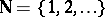of all natural numbers, together with the operations of addition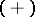and multiplication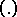, forms the natural number system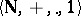. In this system, both binary operations are associative and commutative and satisfy the distributivity law; 1 is the neutral element for multiplication, i.e.for any natural number; there is no neutral element for addition, and, moreover,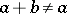for any natural numbers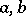. Finally, the following condition, known as the axiom of induction, is satisfied. Any subset of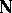that contains 1 and, together with any elementalso contains the sum, is necessarily the whole of. See Natural sequence; Arithmetic, formal.